# Arrhenius Acid: Definition & Examples

Lesson Transcript
Instructor: Nissa Garcia

Nissa has a masters degree in chemistry and has taught high school science and college level chemistry.

Arrhenius acid is a compound that raises the concentration of protons in an aqueous by adding hydrogen ions. Learn the significance of these acids through examples of their chemical reactivity. Updated: 11/02/2021

## What Is an Arrhenius Acid?

What can make a solution be classified as an acid? There are many definitions from different scientists as to what an acid is. In 1884, the Swedish scientist named Svante Arrhenius came up with the definition of an acid, which is known as the Arrhenius acid.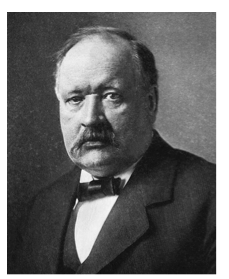Let us imagine an aqueous solution, which is a solution containing water. According to Svante Arrhenius, an acid is a compound that, when in an aqueous solution, increases the number of hydrogen ions (H+), which is a proton. This definition became known as the definition for an Arrhenius acid.

In the chemical equation below, it shows that when the acid, hydrochloric acid (HCl) is dissolved in water, the hydrogen (H) atom and the chlorine (Cl) atom dissociate from each other, and so, the concentration of H+ in an aqueous solution increases due to the release of H+ ions from HCl. The Arrhenius acid is HCl.

Equation 1 below shows the chemical compounds involved in the chemical reaction, and equation 2 shows the structure of these chemical compounds so you can visualize how the H and Cl atoms dissociate from each other in HCl.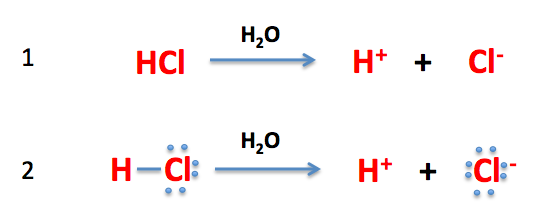When an Arrhenius acid is in water, the acid releases hydrogen ions (H+), which are protons, in water, so it protonates water. When water is protonated, it results in the production of hydronium (H3O+) ions. In the chemical equation shown, it shows that the Arrhenius acid, HCl, protonates water (H2O). As a result, the hydrogen atom from HCl transfers to H2O, and this results in the hydronium ion (H3O+) and the chlorine ion (Cl-). Equation 1 below shows the chemical compounds involved in the chemical reaction, and equation 2 shows the structure of these chemical compounds so you can visualize how the acid protonates water.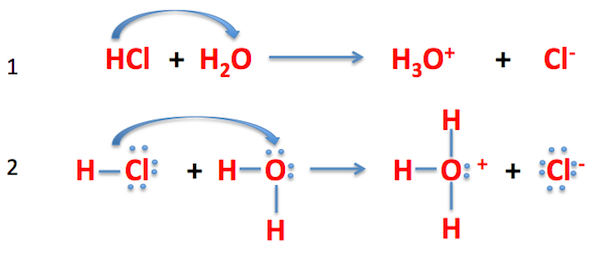Hydrogen (H+) and hydronium (H3O+) ions, in the case of Arrhenius acids, are interchangeable terms. So, we can say that an Arrhenius acid is a compound that increases the hydrogen (H+) or hydronium (H3O+) concentration in an aqueous solution, making the solution more acidic.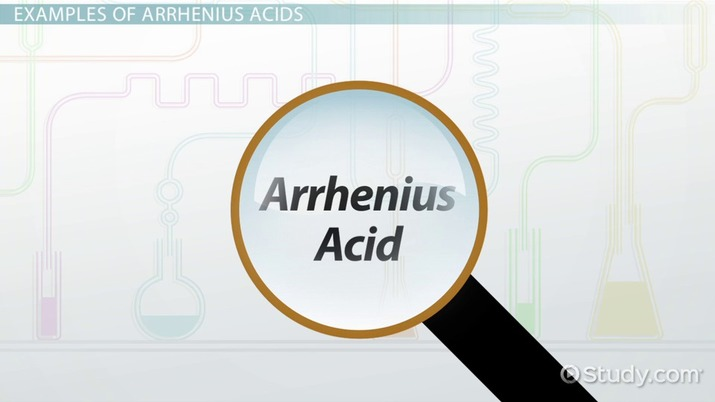An error occurred trying to load this video.

Try refreshing the page, or contact customer support.

Coming up next: Buffer System in Chemistry: Definition & Overview

### You're on a roll. Keep up the good work!

Replay
Your next lesson will play in 10 seconds
• 0:00 What is Arrhenius Acid?
• 2:25 Examples of Arrhenius Acids
• 4:30 Lesson Summary
Save Save

Want to watch this again later?

Timeline
Autoplay
Autoplay
Speed Speed

## Examples of Arrhenius Acids

How do we know if a chemical compound is an Arrhenius acid or not? Arrhenius acids can be binary acids, which are made up of a hydrogen (H) atom and another nonmetallic element, and oxoacids, which are acids made of hydrogen (H), oxygen (O) and at least one other atom.

If we look at the periodic table, binary acids can be formed between hydrogen and elements from group 16 and 17. In the illustration below, the elements that are boxed in red are the ones that typically bond with hydrogen to form binary acids. For instance, hydrogen (H) bonds with fluorine (F) to form the binary acid hydrofluoric acid (HF).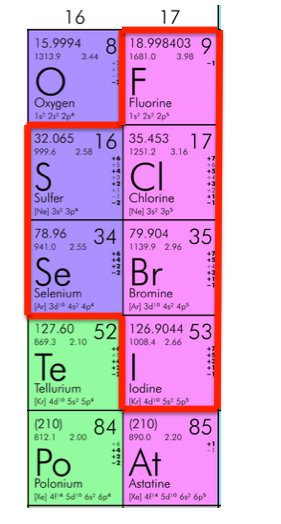To unlock this lesson you must be a Study.com Member.

## Practice:Arrhenius Acid: Definition & Examples Quiz

1/5 completed

### Which of the following are the correct products for the following reaction?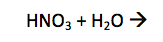Create Your Account To Take This Quiz

As a member, you'll also get unlimited access to over 84,000 lessons in math, English, science, history, and more. Plus, get practice tests, quizzes, and personalized coaching to help you succeed.

It only takes a few minutes to setup and you can cancel any time.

### Register to view this lesson

Are you a student or a teacher?